Search

Exponential Functions: Graphing (page 3 of 5)

Sections: Introduction, Evaluation, Graphing, Compound interest, The natural exponential• Graph h(x) = ( 1/3 )x.

 I will compute the same points as previously: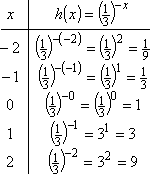Then the graph is: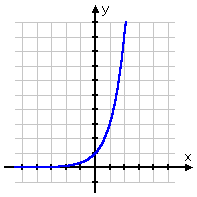So y = ( 1/3 )x does indeed model growth. For the record, however, the base for exponential functions is usually greater than 1, so growth is usually in the form "3x" (that is, with a "positive" exponent) and decay is usually in the form "3x" (that is, with a "negative" exponent).

Every once in a while they'll give you a more-complicated exponential function to deal with:

• Graph y = ex2.

 I will compute some plot-points, as usual: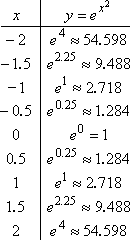Note that, for graphing, the decimal approximations are more useful than the "exact" forms. For instance, it is hard to know where "e2.25" should be plotted, but it's easy to find where "9.488" goes. Note also that I calculated more than just whole-number points. The exponential function grows way too fast for me to use a wide range of x-values (I mean, look how big y got when x was only 2). Instead, I had to pick some in-between points in order to have enough reasonable dots for my graph.

 Now I plot the points, and draw my graph:     Copyright © Elizabeth Stapel 2002-2011 All Rights Reserved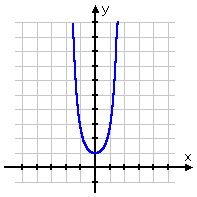The above function did involve an exponential, but was not in the "usual" exponential form (since the power was not linear, but quadratic). However, usually you'll get the more-standard form, with a greater-than-one base, perhaps multiplied by some constant, and a linear exponent. Note that the graphs all look roughly the same; they may have been moved up or down, flipped upside-down, shifted left or right, etc, etc, but they all pretty much have the same shape: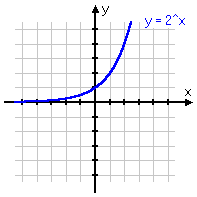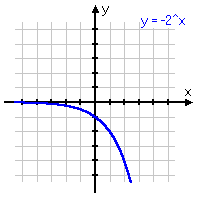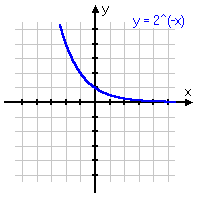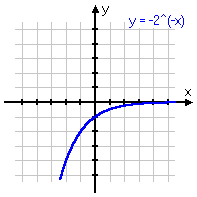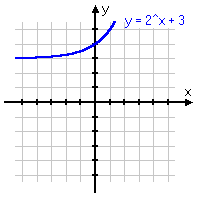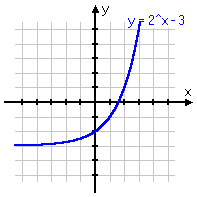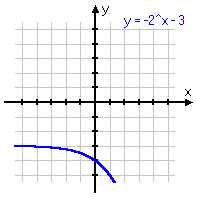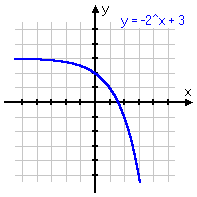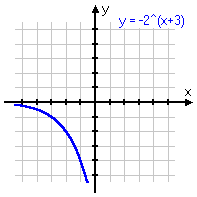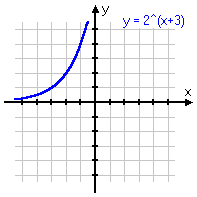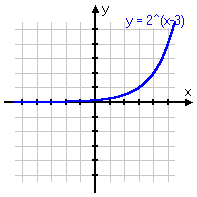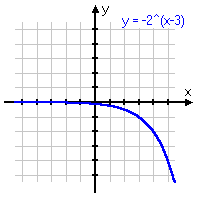<< Previous  Top  |  1 | 2 | 3 | 4 | 5  |  Return to Index  Next >>

 Cite this article as: Stapel, Elizabeth. "Exponential Functions: Graphing." Purplemath. Available from     https://www.purplemath.com/modules/expofcns3.htm. Accessed [Date] [Month] 2016

Study Skills Survey

Tutoring from Purplemath
Find a local math tutor

 Copyright © 2021  Elizabeth Stapel   |   About   |   Terms of Use   |   Linking   |   Site Licensing Contact Us